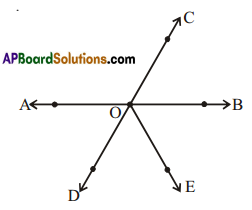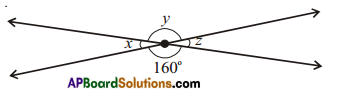# HSSlive: Plus One & Plus Two Notes & Solutions for Kerala State Board

## AP Board Class 7 Maths Chapter 4 Lines and Angles Ex 6 Textbook Solutions PDF: Download Andhra Pradesh Board STD 7th Maths Chapter 4 Lines and Angles Ex 6 Book AnswersAP Board Class 7 Maths Chapter 4 Lines and Angles Ex 6 Textbook Solutions PDF: Download Andhra Pradesh Board STD 7th Maths Chapter 4 Lines and Angles Ex 6 Book Answers

## Andhra Pradesh State Board Class 7th Maths Chapter 4 Lines and Angles Ex 6 Books Solutions

 Board AP Board Materials Textbook Solutions/Guide Format DOC/PDF Class 7th Subject Maths Chapters Maths Chapter 4 Lines and Angles Ex 6 Provider Hsslive

2. Click on the Andhra Pradesh Board Class 7th Maths Chapter 4 Lines and Angles Ex 6 Answers.
3. Look for your Andhra Pradesh Board STD 7th Maths Chapter 4 Lines and Angles Ex 6 Textbooks PDF.
4. Now download or read the Andhra Pradesh Board Class 7th Maths Chapter 4 Lines and Angles Ex 6 Textbook Solutions for PDF Free.

## AP Board Class 7th Maths Chapter 4 Lines and Angles Ex 6 Textbooks Solutions with Answer PDF Download

Find below the list of all AP Board Class 7th Maths Chapter 4 Lines and Angles Ex 6 Textbook Solutions for PDF’s for you to download and prepare for the upcoming exams:

Question 1.
Name two pairs of vertically opposite angles in the figure.Solution:
Vertically opposite angles are
∠AOC, ∠BOD and ∠BOC, ∠AOD

Question 2.
Find the measure of x, y and z without actually measuring them.Solution:
From the figure
∠y and 160° are vertically opposite angles and hence equal.
∴ ∠y=1600
Also ∠x = ∠z and (. vertically opposite angles)
x + 160° = 180° (Linear pair of angles)
∴ ∠x = 180° – 160° = 20°
∠z = 20° (∵ ∠x, ∠z are vertically opposite)
∴ x = 20°
y = 160°
z = 20°

Question 3.
Give some examples of vertically opposite angles in your surroundings.
Solution:
i) Angles between legs of a folding cot/scamp cot.
ii) Angles between legs of a folding chair.
iii) Angles between plates of a scissors.

## Andhra Pradesh Board Class 7th Maths Chapter 4 Lines and Angles Ex 6 Textbooks for Exam Preparations

Andhra Pradesh Board Class 7th Maths Chapter 4 Lines and Angles Ex 6 Textbook Solutions can be of great help in your Andhra Pradesh Board Class 7th Maths Chapter 4 Lines and Angles Ex 6 exam preparation. The AP Board STD 7th Maths Chapter 4 Lines and Angles Ex 6 Textbooks study material, used with the English medium textbooks, can help you complete the entire Class 7th Maths Chapter 4 Lines and Angles Ex 6 Books State Board syllabus with maximum efficiency.

## FAQs Regarding Andhra Pradesh Board Class 7th Maths Chapter 4 Lines and Angles Ex 6 Textbook Solutions

#### Can we get a Andhra Pradesh State Board Book PDF for all Classes?

Yes you can get Andhra Pradesh Board Text Book PDF for all classes using the links provided in the above article.

## Important Terms

Andhra Pradesh Board Class 7th Maths Chapter 4 Lines and Angles Ex 6, AP Board Class 7th Maths Chapter 4 Lines and Angles Ex 6 Textbooks, Andhra Pradesh State Board Class 7th Maths Chapter 4 Lines and Angles Ex 6, Andhra Pradesh State Board Class 7th Maths Chapter 4 Lines and Angles Ex 6 Textbook solutions, AP Board Class 7th Maths Chapter 4 Lines and Angles Ex 6 Textbooks Solutions, Andhra Pradesh Board STD 7th Maths Chapter 4 Lines and Angles Ex 6, AP Board STD 7th Maths Chapter 4 Lines and Angles Ex 6 Textbooks, Andhra Pradesh State Board STD 7th Maths Chapter 4 Lines and Angles Ex 6, Andhra Pradesh State Board STD 7th Maths Chapter 4 Lines and Angles Ex 6 Textbook solutions, AP Board STD 7th Maths Chapter 4 Lines and Angles Ex 6 Textbooks Solutions,
Share: